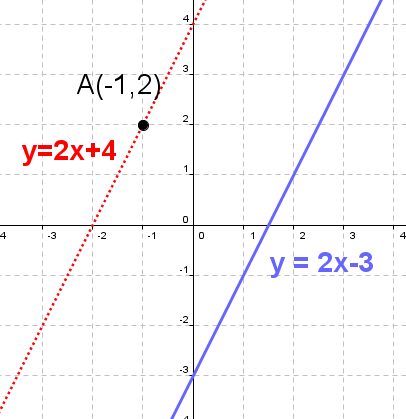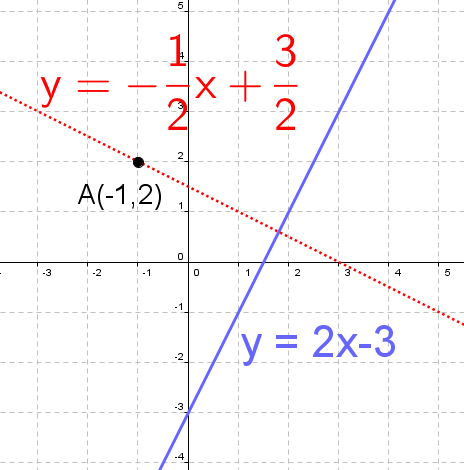Math Calculators, Lessons and Formulas

It is time to solve your math problem

mathportal.org

# Parallel and perpendicular line calculator

This calculator find and plot equations of parallel and perpendicular to the given line and passes through given point. The calculator will generate a step-by-step explanation on how to obtain the result.

Parallel and Perpendicular Line calculator
show help ↓↓ examples ↓↓
 Parallel line through the given point Perpendicular line through the given point
Hide graph
Hide steps
Find approximate solution
working...
examples
example 1:ex 1:
Find the equation of the line that is parallel to $2x + y - 2 = 0$ and passes though the point $( 3, 1 )$.
example 2:ex 2:
Find the equation of the line that is perpendicular to $y = 2x - 5$ and passes though the point $\left( -\frac{2}{3}, -\frac{1}{4} \right)$.

## How to find line through a point parallel to a given line ?

Equation of the line that passes through the point $A(x_0, y_0)$ and is parallel to the line $y = mx + b$ is:

$${\color{blue}{ y - y_0 = m(x-x_0) }}$$

Example:Find the equation of the line that passes through the point $A(-1, 2)$ and is parallel to the line $y = 2x - 3$

Solution:

In this example we have: $x_0 = -1,~~ y_0 = 2,~~ m = 2$. So we have:

\begin{aligned} y - y_0 & = m(x-x_0) \\ y - 2 & = 2(x-(-1)) \\ y - 2 & = 2x + 2 \\ y & = 2x + 2 + 2 \\ {\color{blue}{ y }} & {\color{blue}{ = 2x + 4}} \end{aligned}

Note : If you want to solve this problem using above calculator, you need to rewrite line equation in general form ( $2x - y - 3 = 0$ )

## How to find line through a point perpendicular to a given line ??

Equation of the line that passes through the point $A(x_0, y_0)$ and is perpendicular to the line $y = mx + b$ is:

$${\color{blue}{ y - y_0 = -\frac{1}{m}(x-x_0) }}$$

Example:

Find the equation of the line that passes through the point $A(-1, 2)$ and is perpendicular to the line $y = 2x - 3$

Solution:In this example we have: $x_0 = -1,~~ y_0 = 2,~~ m = 2$. So we have:

\begin{aligned} y - y_0 & = -\frac{1}{m}(x-x_0) \\ y - 2 & = -\frac{1}{2}(x-(-1)) \\ y - 2 & = -\frac{1}{2}(x + 1) \\ (y - 2)\cdot{\color{red}{2}} & = -\frac{1}{2}\cdot{\color{red}{2}}(x + 1) \\ 2(y - 2) & = -(x + 1)\\ 2y - 4 & = -x - 1\\ 2y & = -x + 3\\ {\color{blue}{ y }} & = {\color{blue}{-\frac{1}{2}x + \frac{3}{2} }} \end{aligned}

Note : If you want to solve this problem using above calculator, you need to rewrite line equation in general form ( $2x - y - 3 = 0$ )

Search our database of more than 200 calculators